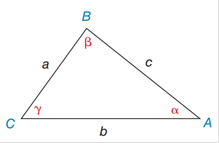Chapter 11.4, Problem 1E### Elementary Geometry for College St...

6th Edition
Daniel C. Alexander + 1 other
ISBN: 9781285195698

#### Solutions

Chapter
Section### Elementary Geometry for College St...

6th Edition
Daniel C. Alexander + 1 other
ISBN: 9781285195698
Textbook Problem
1 views

# In Exercises 1 and 2, use the given information to find an expression for the area of Δ A B C . Give the answer in a form such as A = 1 2 ( 3 ) ( 4 )  sin 32 ∘ . See the figure for Exercises 1- 8.a) a = 5 ,   b = 6 ,  and  γ = 78 ∘ b) a = 5 ,   b = 7 ,   α = 36 ∘  and  β = 88 ∘Exercises 1-8

To determine

a)

To find:

The area of the ABC in the form of A=12(a)(b) sin γ

If a=5, b=6, and γ=78

Explanation

1) Formula:

The area of an acute triangle equals one-half the product of the lengths of two sides and the sine of the included angle.

The area of the triangle ABC is given by,

A=12absinγ

2) Calculation:

Given,

In triangle ABC,

a=5b=6 andγ=78

The area of the triangle ABC A=12absinγ

To determine

b)

To find:

The area of the ABC in the form of A=12(a)(b) sin β

If a=5, b=7, α=36 and β=88

### Still sussing out bartleby?

Check out a sample textbook solution.

See a sample solution

#### The Solution to Your Study Problems

Bartleby provides explanations to thousands of textbook problems written by our experts, many with advanced degrees!

Get Started

#### What are the two requirements for a random sample?

Statistics for The Behavioral Sciences (MindTap Course List)

#### Rationalize the denominator: a. 2x3y b. xx4

Applied Calculus for the Managerial, Life, and Social Sciences: A Brief Approach

#### Solve the equations in Exercises 126. x4x+1xx1=0

Finite Mathematics and Applied Calculus (MindTap Course List)

#### Solve for x: e2x1 = 10. a) 12(1+10e) b) 3 + ln 10 c) 2 + ln 10 d) 1+ln102

Study Guide for Stewart's Single Variable Calculus: Early Transcendentals, 8th